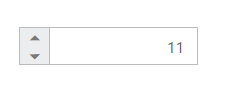# RTL Support

The NumericTextBox provides RTL (Right-To-Left) support. The alignment of NumericTextBox can be changed from Left-To-Right into Right-To-Left.

## Enable RTL

The following steps explain the implementation of enableRTL in NumericTextBox .

In the HTML page set the corresponding <input> elements for rendering NumericTextBox control.

• html
• ``<input id="numeric" type="text" />``
• javascript
• ``````/// <reference path="tsfiles/jquery.d.ts" />
/// <reference path="tsfiles/ej.web.all.d.ts" />
module EditorComponent {
\$(function () {
var number = new ej.NumericTextbox(\$("#numeric"), {
value: 11,
enableRTL: true
});
});
}``````

The output for NumericTextBox when enableRTL is “true” is as follows.